# GMAT Math : DSQ: Understanding work problems

## Example Questions

### Example Question #1 : Dsq: Understanding Work Problems

Clara spentof her salary on rent andof the rest on clothes. How much did she have left after paying the rent and buying the clothes?

(1) Her salary was $3000 (2) She spent$1400 on rent and clothes

Statements (1) and (2) TOGETHER are NOT sufficient.

Statement (1) ALONE is sufficient, but statement (2) alone is not sufficient.

D. EACH statement ALONE is sufficient.

BOTH statements TOGETHER are sufficient, but NEITHER statement ALONE is sufficient.

Statement (2) ALONE is sufficient, but statement (1) alone is not sufficient.

D. EACH statement ALONE is sufficient.

Explanation:

For statement (1), she spent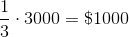on rent, and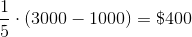on clothes.

So she spent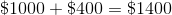in total.

Therefore, she has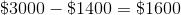left.

For statement (2), we can set Clara’s salary to be, then we have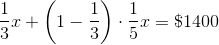Then simplify the equation: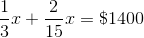Therefore,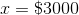.

Now we can just follow what we did for the first statement to calculate the money he had left.

### Example Question #2 : Dsq: Understanding Work Problems

Steve can paint his greenhouse in 4 hours 40 minutes minutes, working alone; his brother Phil can do the same job in 6 hours, working alone. If they work together, to the nearest minute, how long will it take them?

2 hours 42 miutes

3 hours

2 hours 54 minutes

2 hours 38 minutes

2 hours 22 minutes

2 hours 38 minutes

Explanation:

Think of this in terms of "greenhouses per minute", not "minutes per greenhouse". Converting hours and minutes to just minutes, Steve can paint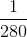greenhouses per minute; Phil can paint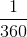greenhouses per minute.

If we letbe the time in minutes that it takes to paint the greenhouse, then Steve and Phil paint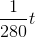and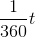greenhouses, respectively; since one greenhouse total is painted, then we can add the labor and set up this equation: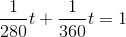Simplify and solve: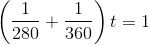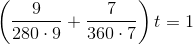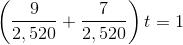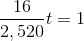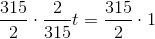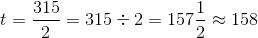or 2 hours, 38 minutes.

### Example Question #1 : Work Problems

A large water tower can be emptied by opening one or both of two drains of different sizes. On one occasion, both drains were opened at the same time. How long did it take to empty the water tower?

Statement 1: Alone, the larger drain can empty the tower in three hours.

Statement 2: The smaller drain can empty water at 75% of the rate at which the larger drain does.

BOTH statements TOGETHER are sufficient to answer the question, but NEITHER statement ALONE is sufficient to answer the question.

Statement 1 ALONE is sufficient to answer the question, but Statement 2 ALONE is NOT sufficient to answer the question.

Statement 2 ALONE is sufficient to answer the question, but Statement 1 ALONE is NOT sufficient to answer the question.

EITHER statement ALONE is sufficient to answer the question.

BOTH statements TOGETHER are insufficient to answer the question.

BOTH statements TOGETHER are sufficient to answer the question, but NEITHER statement ALONE is sufficient to answer the question.

Explanation:

A work problem is actually a rate problem in disguise.

If you know that an object working alone can do a job inhours, then you know that the object works at a rate of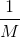jobs per hour. Afterhours, the object accomplishes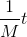of a job. Similarly, the other object working alone does a job inhours, and therefore does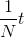of a job. Together, the objects do one whole job, so solve this equation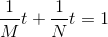for.

Statement 1 alone gives us half the picture;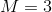, butis unknown.

Statement 2 alone tells us that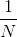is 75% of. Butis unknown.

From Statement 1 and 2 together, we knowis 75% of- this allows us to calculate:

75% ofis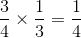, so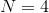. Since we have bothand, we have the complete equation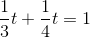and we can calculate.

### Example Question #4 : Dsq: Understanding Work Problems

A large water tower can be emptied by opening one or both of two drains of different sizes. On one occasion, both drains were opened at the same time. How long did it take to empty the water tower?

Statement 1: Alone, the smaller drain can empty the tower in three hours.

Statement 2: Alone, the larger drain can empty the tower in two hours.

BOTH statements TOGETHER are sufficient to answer the question, but NEITHER statement ALONE is sufficient to answer the question.

EITHER statement ALONE is sufficient to answer the question.

Statement 1 ALONE is sufficient to answer the question, but Statement 2 ALONE is NOT sufficient to answer the question.

Statement 2 ALONE is sufficient to answer the question, but Statement 1 ALONE is NOT sufficient to answer the question.

BOTH statements TOGETHER are insufficient to answer the question.

BOTH statements TOGETHER are sufficient to answer the question, but NEITHER statement ALONE is sufficient to answer the question.

Explanation:

A work problem is actually a rate problem in disguise.

If you know that an object working alone can do a job inhours, then you know that the object works at a rate ofjobs per hour. Afterhours, the object accomplishesof a job. Similarly, the other object working alone does a job inhours, and therefore doesof a job. Together, the objects do one whole job, so solve this equationfor.

Statement 1 alone tells us that, and Statement 2 alone tells us that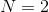.

Therefore, each statement alone gives us only half the picture, but together, they give us the equation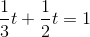,

which can be solved to yield the answer.

### Example Question #5 : Dsq: Understanding Work Problems

Three brothers - David, Eddie, and Floyd - mow a lawn together, starting at the same time. How long will it take them to finish?

Statement 1: Working alone, David can mow the lawn in four hours.

Statement 2: Working together, but without David, Eddie and Floyd can mow the lawn in three hours.

EITHER statement ALONE is sufficient to answer the question.

BOTH statements TOGETHER are sufficient to answer the question, but NEITHER statement ALONE is sufficient to answer the question.

BOTH statements TOGETHER are insufficient to answer the question.

Statement 2 ALONE is sufficient to answer the question, but Statement 1 ALONE is NOT sufficient to answer the question.

Statement 1 ALONE is sufficient to answer the question, but Statement 2 ALONE is NOT sufficient to answer the question.

BOTH statements TOGETHER are sufficient to answer the question, but NEITHER statement ALONE is sufficient to answer the question.

Explanation:

A work problem is actually a rate problem in disguise.

If you know that David working alone can do a job inhours, then you know that the object works at a rate ofjobs per hour. Afterhours, the object accomplishesof a job. Similarly, Eddie and Floyd, working without David, do a job inhours, and therefore doesof a job. Together, the objects do one whole job, so solve this equationfor.

Statement 1 alone tells us that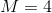, and Statement 2 alone tells us that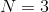; each one alone leaves the other value unknown. However, if both statements are given, the equation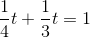can be solved forto yield the correct answer.

### Example Question #6 : Dsq: Understanding Work Problems

Last week, Mrs. Smith, Mrs. Edwards, and Mrs. Hume were able to write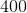invitations to a party in two hours.

Today, Mrs. Smith is sick and cannot help, so Mrs. Edwards, and Mrs. Hume have to work without her. They must writemore invitations to the same party. How long should they take, working together?

Statement 1: Working alone, Mrs. Smith can writeinvitations in one and one-half hours.

Statement 2: Working alone, Mrs. Hume can writeinvitations in one hour.

BOTH statements TOGETHER are insufficient to answer the question.

Statement 1 ALONE is sufficient to answer the question, but Statement 2 ALONE is NOT sufficient to answer the question.

BOTH statements TOGETHER are sufficient to answer the question, but NEITHER statement ALONE is sufficient to answer the question.

EITHER statement ALONE is sufficient to answer the question.

Statement 2 ALONE is sufficient to answer the question, but Statement 1 ALONE is NOT sufficient to answer the question.

Statement 1 ALONE is sufficient to answer the question, but Statement 2 ALONE is NOT sufficient to answer the question.

Explanation:

Since the group is now without the help of Mrs. Smith, we look at Mrs. Smith's contribution to the work as a whole, and the sum of the other two ladies' contribution as a whole; Statement 2, which deals with Mrs. Hume alone, is irrelevant and unhelpful.

Since the three ladies together wrote 400 invitations in 120 minutes, we can infer that they would have spent three-fourths of this time, or 90 minutes, writing 300 invitations.

If Statement 1 is true, then Mrs. Smith, who can write 100 invitations in 90 minutes, would take three times this, or 270 minutes, to write 300 invitations.

A work problem is a rate problem in disguise. Think in terms of "jobs per hour", and take the reciprocal of each "hours per job". The three ladies together would have done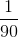job in one hour, and Mrs. Smith alone would have done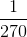job in one hour.

Therefore, in one hour today, the two ladies will do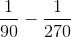jobs, and inhours today, they will do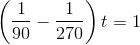job.

Solve forin this equation.

This proves that Statement 1 alone allows us to find the answer - but not Statement 2.